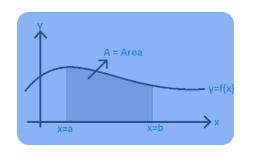# Area under a curve.

Hi ya,

New to KNIME…looking for a way to calculate area under a curve. I just have two columns, X & Y.
Wasn’t able to set up the ROC curve got error no positive values in class column. Only column available in drop down is the x column…

Thanks alot !

@Gimli welcome to the knime forum. You can use

You typically will have to configure the column with the score prediction and the truth with the positive class.

1 Like

Thanks for the response…I have two columns with values for X n Y axis…It automatically selects the x axis
as the class column. A warning shows up that says “Column X axis contains no possible positive values”.
The values start at 0 and increment by 1 …not sure what that means.

thanks alot

@Gimli question is what do you want to measure. the nodes mentioned would have a prediction (score) that typically rund between 0 and 1 and a (binary) variable of 1 and 0 (true/false) that would be the ground truth about what your model tried to predict (taken from the test or validation data). You can read more about that here:

If you have other constellations or you have a regression model other statistics / graphics would be needed.

1 Like

Hi ya,

I just want to measure the area under the curve created by the X n Y values if Im explaining that correctly…not a prediction? a definite integral…integrate y = f(x) between the limits of a and b@Gimli OK I hope I got what you want. I converted the Python code from two Stackoverflow threads (1, 2) into a small KNIME workflow. You might want to check if you could adapt that.

You would enter the data and the variables and the numeric borders

The lower part of the workflow would just use the x-axis min and max as a default. You might be able to tweak the design of the y-axis.

kn_example_python_numeric_integral.knwf (341.2 KB)

5 Likes

Wow that’s great!! thanks so much!! Looks like you did alot of work!
Exactly what I was looking for!

2 Likes